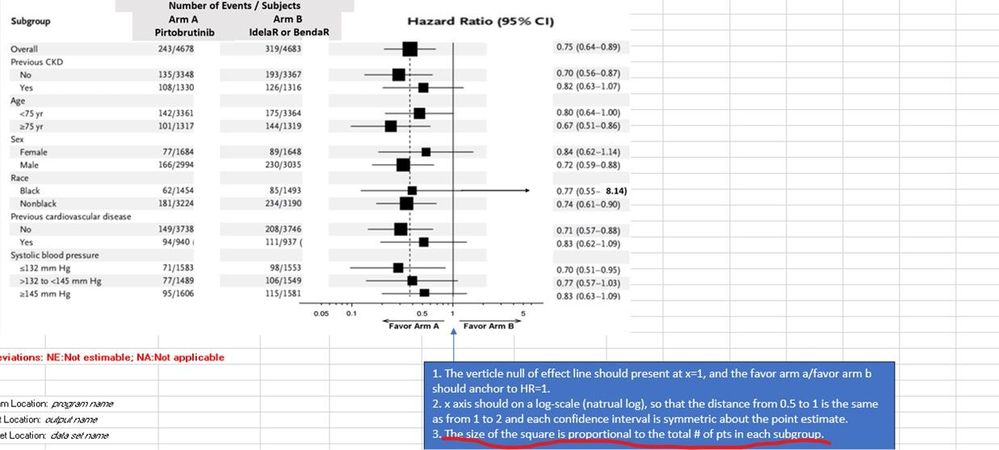## Forest plot with Proc Template

Hello everyone,

I am working on a forest plot and using Proc Template to generate it. I have an interesting requirement, which is to make the size of the hazard ratio symbol proportional to the number of subjects in each group. I am wondering if there is a way to do this. Any suggestions would be greatly appreciated.

``````proc template;
define statgraph Forest;
dynamic  _color_ _headercolor ystart ystep ystop label ;
begingraph/designheight=2.6in;;
layout lattice/ columns=5 columnweights=(0.50 0.20 0.20 0.45 0.45); *columnweights=(0.85 0.40 0.50 0.70 0.60);

sidebar / align=top;
layout lattice / rows=3 columns=3 columnweights=(0.30 0.55 .70)
entry textattrs=(size=8 weight=bold) halign=left "                   ";
entry textattrs=(size=8 weight=bold) halign=center "Number of Events/ Subjects";
entry textattrs=(size=8 weight=bold) halign=left "                   ";
entry textattrs=(size=8 weight=bold) halign=left "Subgroup";
entry textattrs=(size=8 weight=bold) halign=center "   ARM A                   ARM B";
entry textattrs=(size=8 weight=bold) halign=right "Hazard Ratio (95% CI)   .";
entry textattrs=(size=8 weight=bold) halign=left label;
entry textattrs=(size=8 weight=bold) halign=center "           Pirtobrutinib            IdelaR or BendaR";
entry textattrs=(size=8 weight=bold) halign=left "  ";

endlayout;
endsidebar;
/*--First Subgroup column, shows only the Y2 axis--*/

layout overlay / walldisplay=none
xaxisopts=(offsetmin=0 offsetmax=0 display=none linearopts=(viewmin=0 viewmax=20))
yaxisopts=(offsetmin=0.03 offsetmax=0.03 reverse=true display=none tickvalueattrs=(weight=bold));
highlowplot y=n low=zero high=zero / highlabel=param  lineattrs=(thickness=0 )
labelattrs=(size=8 weight=bold);
highlowplot y=n low=zero high=one / highlabel=paramcd lineattrs=(thickness=0) labelattrs=(size=8);
endlayout;
/*--Second Subgroup column, shows only the Y2 axis--*/
layout overlay / xaxisopts=(offsetmin=0 offsetmax=0 display=none) CYCLEATTRS=FALSE
yaxisopts=(offsetmin=0.03 offsetmax=0.03 reverse=true display=none) walldisplay=none;
scatterplot y=N x=arma / markercharacter=arm_a
markercharacterattrs=graphvaluetext ;
endlayout;

layout overlay / xaxisopts=(offsetmin=0 offsetmax=0 display=none) CYCLEATTRS=FALSE
yaxisopts=(offsetmin=0.03 offsetmax=0.03 reverse=true display=none) walldisplay=none;
scatterplot y=N x=armb / markercharacter=arm_b
markercharacterattrs=graphvaluetext ;
endlayout;

/*--Fifth column showing odds ratio graph--*/
layout overlay / xaxisopts=( /*display=none*/ label='<-Favor Arm A-Favor Arm B->'
linearopts=(tickvaluepriority=true tickvaluesequence=(start=ystart increment=ystep end=ystop) ))
yaxisopts=(offsetmin=0.03 offsetmax=0.03 reverse=true display=none) walldisplay=none CYCLEATTRS=FALSE;
scatterplot y=n x=HAZARDRATIO/ xerrorlower=HRLOWERCL xerrorupper=HRUPPERCL
markerattrs=(symbol=circlefilled size=10);
referenceline x=1.0;
endlayout;

/*--Sixth column showing hazard ratio and CI --*/
layout overlay / xaxisopts=(offsetmin=0 offsetmax=0 display=none label='<-Favor Arm A-Favor Arm B->')
yaxisopts=(offsetmin=0.03 offsetmax=0.03 reverse=true display=none) walldisplay=none CYCLEATTRS=FALSE;
scatterplot y=N x=zero / markercharacter=HZci_
markercharacterattrs=graphvaluetext;
endlayout;
endlayout;
endgraph;
end;
run;``````2 REPLIES 2

## Re: Forest plot with Proc Template

What does the input data set look like? And an example call to Proc SGRENDER so we know how you are thinking of using this.

I strongly suspect you will have to provide that ratio value in the data to have a chance of getting this idea to work.

Then  you could use the EVAL(< your ratio variable goes here>) in the Size=  suboption of a Markerattrs= option. Unless you have a variable with counts. I can't tell what any of your variables actually are just from syntax. If you have the count variables then it would be Eval( somevar/othervar )  . You may need to through in a fixed multiplier to scale the ratios to a desired dimension size but no idea what that might need to be without data. I suspect getting consistent scaling is likely to be in a step outside of the GTL though.Ksharp
Super User

## Re: Forest plot with Proc Template

Discussion stats
• 2 replies
• 177 views
• 0 likes
• 3 in conversation